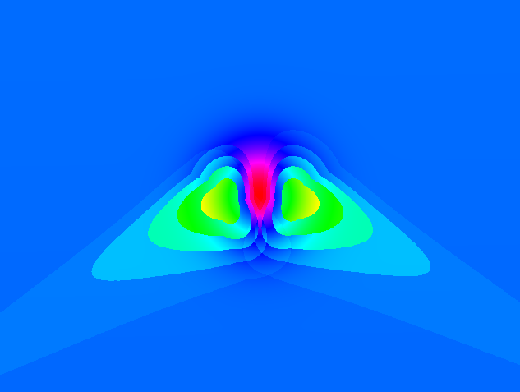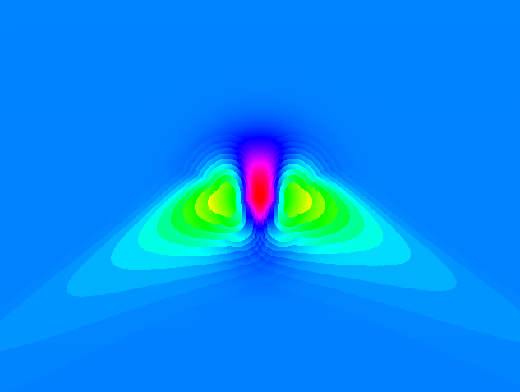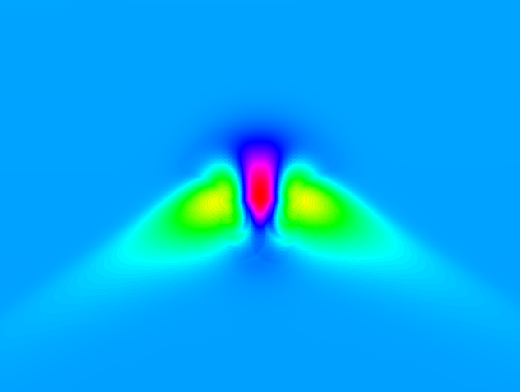# Difference between revisions of "R.sun"

## Tips

The speed of r.sun is much higher if r.horizon is used first and the resulting maps are given as input to r.sun. Background: the horizon needs to be computed only one time before, not in every step within r.sun. See the example at the end of the r.sun help page.

### Testing

Create an artificial surface containing a Gaussian mound:

```r.surf.volcano out=gauss method=gaussian kurtosis=1
```

Overlay some 200m contours to show underlying topography:

```r.contour in=gauss out=gauss_200m_contours step=200
d.vect gauss_200m_contours color=white
```

Set map's color table to highlight detail:

```r.colors rad_test.day355.beam col=bcyr -e
```

### Time step

The following three images demonstrate the effects of using different time step parameters.

```r.sun -s elevin="gauss" glob_rad="rad.global.30minT" day=180 step=0.5
```Default 30 minute time step over a Gaussian mound.

```r.sun -s elevin="gauss" glob_rad="rad.global.15minT" day=180 step=0.25
```15 minute time step over a Gaussian mound.

```r.sun -s elevin="gauss" glob_rad="rad.global.03minT" day=180 step=0.05
```3 minute time step over a Gaussian mound.

The 3 minute time step takes roughly ten times as long to run as the 30 minute timestep.

## Seed maps

Using seed maps can greatly speed up processing. This is especially important if you will batch process e.g. for every day of the year.

• aspin= and slopein= options: create slope and aspect maps with the r.slope.aspect module.
Caution: currently buggy. See trac #498.
Estimated speedup:  ?%.

• horizon= and horizonstep= options: Pre-calculate horizon shadows by creating a series of horizon rasters with the r.horizon module.
Results not as smooth as without using this option?? See trac #498.
Estimated speedup:  ?%.

• latin= option: Pre-calculate latitudes for each raster cell.

The following script will create a raster containing latitude as the raster value:

``` # create latitude map (WGS84)
g.region rast=elevation.dem
r.mapcalc one=1
r.out.xyz one | \
cut -f1,2 -d'|' | \
m.proj -oed --quiet | \
sed -e 's/[ \t]/|/g' | \
cut -f1,2,4 -d'|' | \
r.in.xyz in=- out=elevation.lat
g.remove one
```
Estimated speedup:  ?%.
See trac #498.

## ToDo

• Add support for OpenMP parallelization